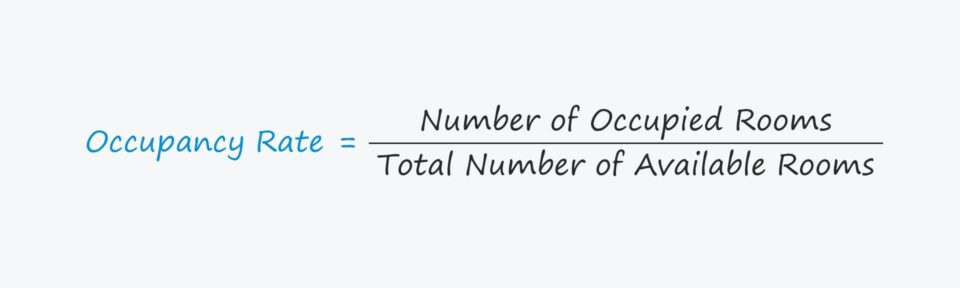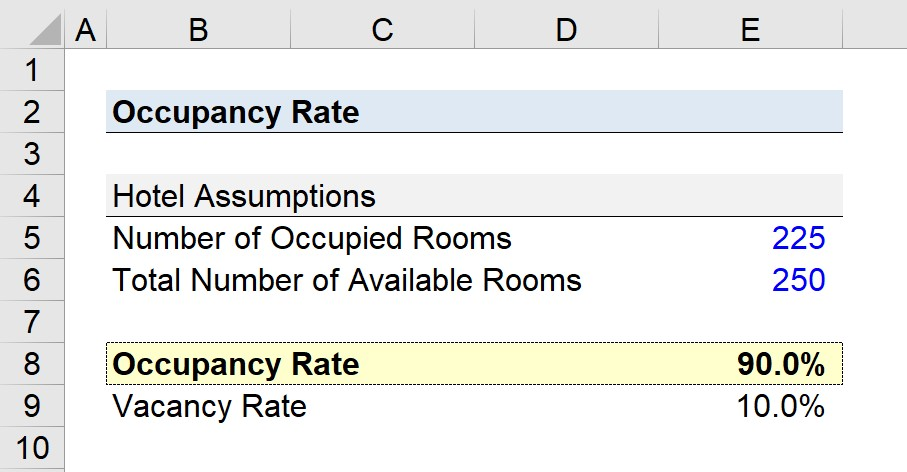Welcome to Wall Street Prep! Use code at checkout for 15% off.# Occupancy Rate

Guide to Understanding the Occupancy Rate Concept## How to Calculate Occupancy Rate?

The occupancy rate is a real estate metric that measures the number of occupied rental units at a specific point in time, relative to the number of rental units available, expressed as a percentage.

In particular, the occupancy rate is a key performance indicator (KPI) within the hospitality sector, namely hotels, since the metric quantifies the proportion of a rental property that is actually being utilized (and generating cash flow).

Common examples of industries where the occupancy rate (or vacancy rate) is an important determinant of revenue include the following examples.

• Hospitality Sector, e.g. Hotel Properties
• Apartment Complex
• Hospitals
• Healthcare Assisted Living Facilities
• C2C Rental Platform (i.e. Airbnb)

Since an unoccupied rental unit, such as a hotel room, generates no revenue, a hotel strives to achieve as high occupancy as possible.## Occupancy Rate Formula

The formula for calculating the occupancy at a hotel is as follows.

Occupancy Rate (%) = Number of Occupied Rooms ÷ Total Number of Available Rooms

For example, if a hotel with 100 available rooms currently has 85 rooms booked, the occupancy is 85% on the given day.

• Occupancy Rate (%) = 85 ÷ 100 = 0.85, or 85%

Or, if the hotel has 100 rooms available for rent and 100 of the rooms are occupied, the occupancy rate is 100% – i.e. full occupancy with regard to capacity.

## Occupancy Rate vs. Vacancy Rate: What is the Difference?

In real estate property management, the occupancy rate and vacancy rate are two critical measures to ensure a property generates consistent, long-term cash flow, while mitigating risk.

The formula to calculate the vacancy rate is as follows.

Vacancy Rate = Number of Vacant Units ÷ Total Number of Units

The occupancy and vacancy rate metrics are inversely related, i.e. “two sides of the same coin”.

• Occupancy Rate (%) → The occupancy rate is the proportion of a property’s total units that is rented or occupied at present, expressed as a percentage.
• Vacancy Rate (%) → In contrast, the vacancy rate is the proportion of a property’s total units that are unoccupied, i.e. vacant with no tenants.

If the occupancy rate increases, the vacancy rate decreases, and vice versa.

On the subject of effective property management, the objective of property owners and real estate investors should be to maximize the occupancy rate (and minimize the vacancy rate).

Why? Higher occupancy rates coincide with effective property management, strong market demand, and lower perceived risk.

Given the inverse relationship, the sum of the occupancy rate and vacancy rate must equal 1.0 (or 100%).

Therefore, the vacancy rate can be computed by subtracting the occupancy rate from one.

Vacancy Rate = 1 Occupancy Rate

## What is a Good Occupancy Rate?

The closer a hotel’s occupancy is near full-capacity (100%) – i.e. the full utilization of all available rental units – the closer the hotel is to profit maximization, all else being equal.

But higher occupancy does not necessarily always translate into higher revenue, because other factors, such as the average daily rate (ADR) and the revenue per available room, must also be considered.

For instance, a hotel with 85% occupancy could bring in more revenue than a competitor with 100% occupancy if the former charged sufficiently higher prices.

• Higher Pricing Rates → Lower Occupancy Rates (and Higher Vacancy Rates)
• Lower Pricing Rates → Higher Occupancy Rates (and Lower Vacancy Rates)

Simply put, a hotel with above-market pricing will have a business model that reduces its dependence on near-full capacity occupancy to reach its target revenue.

To maximize revenue generation, hotel owners and renters must understand the trade-off between pricing and occupancy when setting prices.

## Occupancy Rate Calculator

We’ll now move to a modeling exercise, which you can access by filling out the form below.#### Get the Excel Template!Submitting...

## Hotel Occupancy Rate Calculation Example

Suppose a hotel, a property operating in the hospitality sector, has 250 rooms available for customers to book.

On the date of our analysis, the number of occupied rooms is 225, so only 25 rooms are vacant (i.e. unoccupied).

• Number of Occupied Rooms = 225
• Total Number of Available Rooms = 250

What is the occupancy rate of the hotel on this specific date?

Given these assumptions, the occupancy rate is 90%, which we calculated by dividing the number of occupied rooms by the total available rooms.

• Occupancy Rate (%) = 225 ÷ 250 = 90.0%

In conclusion, we can also back-solve the vacancy rate by subtracting the hotel’s occupancy rate from one.

• Vacancy Rate (%) = 1 – 90% = 10.0%Step-by-Step Online Course

### Everything You Need To Master Financial Modeling

Enroll in The Premium Package: Learn Financial Statement Modeling, DCF, M&A, LBO and Comps. The same training program used at top investment banks.

Inline Feedbacks